# Controlled systems

## Overview Controlled Systems

Descripe and classify controlled systems

In order to check and classify the behavior of the controlled systems, the input signal is preferably a voltage change from 0V to 1V. The step response can be used to determine parameters of the controlled system: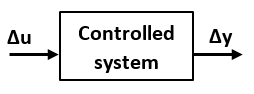Evaluate step response of controlled system

Proportional Controlled systems

Controlled systems with proportional behavior or P-systems have an end value or steady state value and are therefore also referred to as controlled systems. They can be further distinguished in P- systems with and without delay time and dead time:

PT0-system

A controlled system without delay exists when the controlled variable follows the manipulated variable without a measurable time delay and thus suddenly reaches its steady state. In practice, there is practically no pure P-system. Even a transistor has a delay. However, for simplicity, if the time delay is very small or barely measurable, then the reaction can be idealized.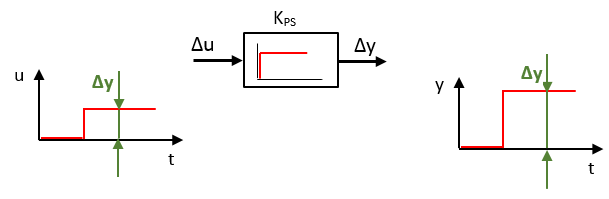Step response and symbol PT0-system

Parameter to describe this system: Transfer coefficient Kps also referred as gain:  KPS = Δx/Δy

P-T1-element (1st order system)

The behavior of a single storage element system (1st order system) can be described by an exponential function: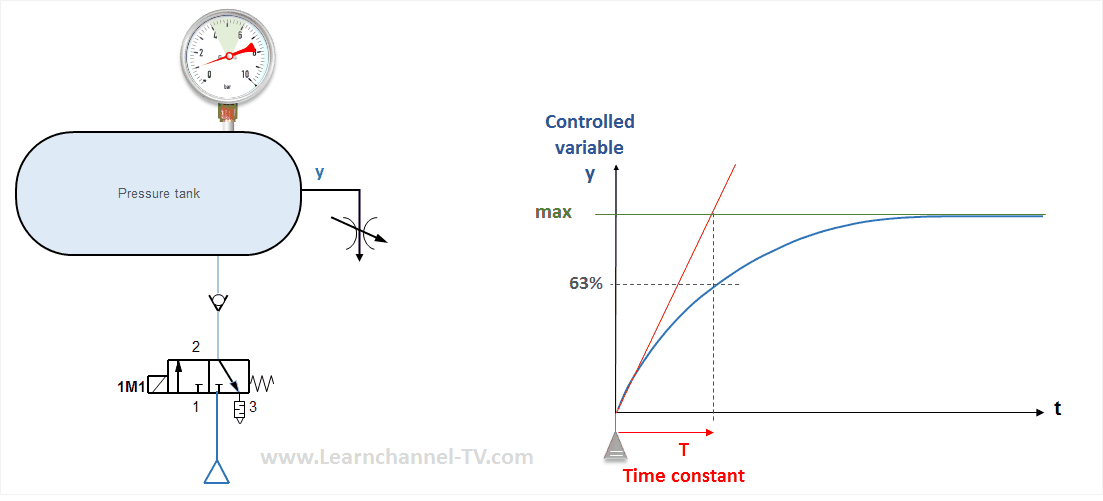P-T1-plant (1st order system)

Parameter: Transfer coefficient Ks  , Time constant T

The e-function is described by:   x = Ks * y * (1 – e –t/T)

The time constant T is a measure for how fast the steady-state condition can be reached. Graphically it can be determined using the slope tangent at the starting point. This tangent cuts the straight line of the maximum value. Now draw a straight line vertically to the time axis.

The time constant is the time that the controlled value x needs to reach 63,2% of the final value (maximum, steady-state). Practically, the steady-state is reached after about 5T.

Multiple storage element ( P-Tn)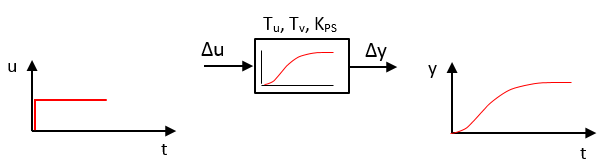step response and symbol of PTn system

If a controlled system has severstep response and symbol PTn-systemal stages which store energy, then you call it a PTn - system. Here you see an example: A tin bath (PT2-system) consisiting of 2 separate elements: the heating resistors and the tin itself - both elements store energy in form of heat.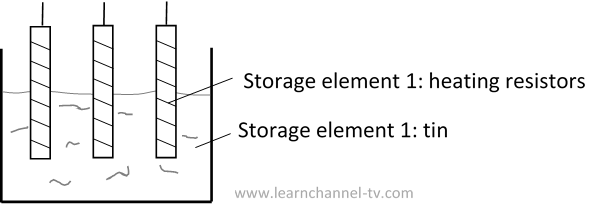Closed-loop control - Multiple storage element ( P-Tn)

How to determine the parameter via the step-response you see here: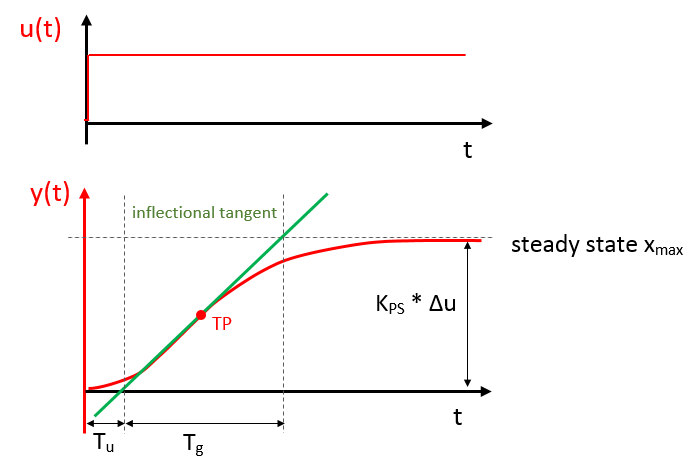Step response of PTn-system

Parameter:      Tg   balancing time,   Tu   delay time